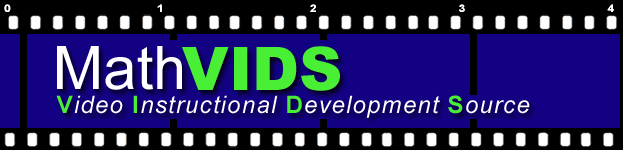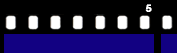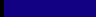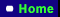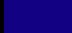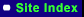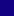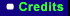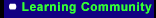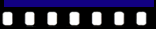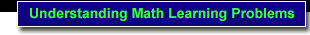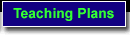Concrete - Representational - Abstract

Sequence of Instruction

 Purpose Description Elements Implementation Impact Videos

Purpose

The purpose of teaching through a concrete-to-representational-to-abstract sequence of instruction is to ensure students truly have a thorough understanding of the math concepts/skills they are learning. When students who have math learning problems are allowed to first develop a concrete understanding of the math concept/skill, then they are much more likely to perform that math skill and truly understand math concepts at the abstract level.

What is it?

• Each math concept/skill is first modeled with concrete materials (e.g. chips, unifix cubes, base ten blocks, beans and bean sticks, pattern blocks).
• Students are provided many opportunities to practice and demonstrate mastery using concrete materials
• The math concept/skill is next modeled at the representational (semi-concrete) level which involves drawing pictures that represent the concrete objects previously used (e.g. tallies, dots, circles, stamps that imprint pictures for counting)
• Students are provided many opportunities to practice and demonstrate mastery by drawing solutions
• The math concept/skill is finally modeled at the abstract level (using only numbers and mathematical symbols)
• Students are provided many opportunities to practice and demonstrate mastery at the abstract level before moving to a new math concept/skill.
• As a teacher moves through a concrete-to-representational-to-abstract sequence of instruction, the abstract numbers and/or symbols should be used in conjunction with the concrete materials and representational drawings (promotes association of abstract symbols with concrete & representational understanding)

What are the critical elements of this strategy?

• Use appropriate concrete objects to teach particular math concept/skill (see Concrete Level of Understanding/Understanding Manipulatives-Examples of manipulatives by math concept area). Teach concrete understanding first.
• Use appropriate drawing techniques or appropriate picture representations of concrete objects (see Representational Level of Understanding/Examples of drawing solutions by math concept area). Teach representational understanding second.
• Use appropriate strategies for assisting students to move to the abstract level of understanding for a particular math concept/skill (see Abstract Level of Understanding/Potential barriers to abstract understanding for students who have learning problems and how to manage these barriers).
• When teaching at each level of understanding, use explicit teaching methods (see the instruction strategy Explicit Teacher Modeling).

How do I implement the strategy?

1. When initially teaching a math concept/skill, describe & model it using concrete objects (concrete level of understanding).
2. Provide students many practice opportunities using concrete objects.
3. When students demonstrate mastery of skill by using concrete objects, describe & model how to perform the skill by drawing or with pictures that represent concrete objects (representational level of understanding).
4. Provide many practice opportunities where students draw their solutions or use pictures to problem-solve.
5. When students demonstrate mastery drawing solutions, describe and model how to perform the skill using only numbers and math symbols (abstract level of understanding).
6. Provide many opportunities for students to practice performing the skill using only numbers and symbols.
7. After students master performing the skill at the abstract level of understanding, ensure students maintain their skill level by providing periodic practice opportunities for the math skills.

How Does This Instructional Strategy Positively Impact Students Who Have Learning Problems?

• Helps passive learner to make meaningful connections
• Teaches conceptual understanding by connecting concrete understanding to abstract math process
• By linking learning experiences from concrete-to-representational-to-abstract levels of understanding, the teacher provides a graduated framework for students to make meaningful connections.
• Blends conceptual and procedural understanding in structured way

Research Support For The Instructional Features Of This Instructional Strategy: Allsopp (1999); Baroody (1987); Butler, Miller, Crehan, Babbit, & Pierce (2003); Harris, Miller, & Mercer (1993);  Kennedy and Tips (1998); Mercer, Jordan, & Miller (1996); Mercer and Mercer (2005); Miller, Butler, & Lee (1998); Miller and Mercer, 1995; Miller, Mercer, & Dillon (1992); Peterson, Mercer, & O'Shea. (1988); Van De Walle (2005); Witzel, Mercer, & Miller (2003).

Create seperate pages

Concrete

What is it?
Understanding manipulatives
Examples of manipulatives (concrete objects) by math concept level
Suggestions for using manipulatives (concrete objects)

What is it?

The concrete level of understanding is the most basic level of mathematical understanding. It is also the most crucial level for developing conceptual understanding of math concepts/skills. Concrete learning occurs when students have ample opportunities to manipulate concrete objects to problem-solve. For students who have math learning problems, explicit teacher modeling of the use of specific concrete objects to solve specific math problems is needed.

Understanding manipulatives (concrete objects)

To use math manipulatives effectively, it is important that you understand several basic characteristics of different types of math manipulatives and how these specific characteristics impact students who have learning problems. As you read about the different types of manipulatives, click on the numbers beside each description to view pictures of these different types of manipulatives.

General types of math manipulatives:

Discrete - those materials that can be counted (e.g. cookies, children, counting blocks, toy cars, etc.).

See examples - 1 | 2 | 3 | 4 |

Continuous - materials that are not used for counting but are used for measurement (e.g. ruler, measuring cup, weight scale, trundle wheel). See example - 1

Suggestions for using Discrete & Continuous materials with students who have learning problems:

Students who have learning problems need to have abundant experiences using discrete materials before they will benefit from the use of continuous materials. This is because discrete materials have defining characteristics that students can easily discriminate through sight and touch. As students master an understanding of specific readiness concepts for specific measurement concepts/skills through the use of discrete materials (e.g. counting skills), then continuous materials can be used.

Types of manipulatives used to teach the Base-10 System/place-value (Smith, 1997):

Proportional - show relationships by size (e.g. ten counting blocks grouped together is ten times the size of one counting block; a beanstick with ten beans glued to a popsicle stick is ten times bigger than one bean).

- Non-linked proportional - single units are independent of each other, but can be "bundled together (e.g. popsicle sticks can be "bundled together in groups of 'tens' with rubber bands; individual unifix cubes can be attached in rows of ten unifix cubes each). See examples - 1 | 2 | 3 |

- Linked proportional - comes in single units as well as "already bundled" tens units, hundreds units, & thousands units (e.g. base ten cubes/blocks; beans & beansticks). Se examples - 1 | 2 | 3 | 4 | 5

Non-proportional
- use units where size is not indicative of value while other characteristics indicate value (e.g. money, where one dime is worth ten times the value of one penny; poker chips where color indicates value of chip; an abacus where location of the row indicates value). A specified number of units representing one value are exchanged for one unit of greater value (e.g. ten pennies for one dime; ten white poker chips for one blue poker chip, ten beads in the first row of an abacus for one bead in the second row). See example - 1

Suggestions for using proportional and non-proportional manipulatives with students who have learning problems:

Students who have learning problems are more likely to learn place value when using proportional manipulatives because differences between ones units, tens units, & hundreds units are easy to see and feel. Due to the very nature of non-proportional manipulatives, students who have learning problems have more difficulty seeing and feeling the differences in unit values.

Examples of manipulatives (concrete objects)

Suggested manipulatives are listed according to math concept/skill area. Descriptions of manipulatives are provided as appropriate. A brief description of how each set of manipulatives may be used to teach the math concept/skill is provided at the bottom of the list for each math concept area. Picture examples of some of the manipulatives for each math concept area can be accessed by clicking on the numbers found underneath the title of each math concept area. This is not meant to be an exhaustive list, but this list does include a variety of common manipulatives. The list includes examples of "teacher-made" manipulatives as well "commercially-made" ones.

Place Value
Multiplication/Division
Positive & Negative Integers
Fractions
Geometry
Beginning Algebra

See examples - 1 | 2 | 3 |

• Colored chips
• Beans
• Unifix cubes
• Golf tees
• Skittles or other candy pieces
• Packaging popcorn
• Popsicle sticks/tongue depressors

Description of use: Students can use these concrete materials to count, to add, and to subtract. Students can count by pointing to objects and counting aloud. Students can add by counting objects, putting them in one group and then counting the total. Students can subtract by removing objects from a group and then counting how many are left.

Place Value Pictures

See examples - 1 | 2 | 3 | 4 | 5 | 6 | 7

• Base 10 cubes/blocks
• Beans and bean sticks
• Popsicle sticks & rubber bands for bundling
• Unifix cubes (individual cubes can be combined to represent "tens")
• Place value mat (a piece of tag board or other surface that has columns representing the "ones," "tens," and "hundreds" place values)

Description of use: Students are first taught to represent 1-9 objects in the "ones" column. They are then taught to represent "10" by trading in ten single counting objects for one object that contains the ten counting objects on it (e.g. ten separate beans are traded in for one "beanstick" - a popsicle stick with ten beans glued on one side. Students then begin representing different values 1-99. At this point, students repeat the same trading process for "hundreds."

Multiplication/Division Pictures

See examples - 1 | 2 | 3 |

• Containers & counting objects (paper dessert plates & beans, paper or plastic cups and candy pieces, playing cards & chips, cutout tag board circles & golf tees, etc.). Containers represent the "groups" and counting objects represent the number of objects in each group. (e.g. 2 x 4 = 8: two containers with four counting objects on each container)
Counting objects arranged in arrays (arranged in rows and columns). Color-code the "outside" vertical column and horizontal row helps emphasize the multipliers

.
Positive & Negative Integers Pictures

See examples - 1

• Counting objects, one set light colored and one set dark colored (e.g. light & dark colored beans; yellow & blue counting chips; circles cut out of tag board with one side colored, etc.).

Description of use: Light colored objects represent positive integers and dark colored objects represent negative integers. When adding positive and negative integers, the student matches pairs of dark and light colored objects. The color and number of objects remaining represent the solution.

Fractions Pictures

See examples - 1 | 2 | 3 |

• Fraction pieces (circles, half-circles, quarter-circles, etc.)
• Fraction strips (strips of tag board one foot in length and one inch wide, divided into wholes, ½'s, 1/3's, ¼'s, etc.
• Fraction blocks or stacks. Blocks/cubes that represent fractional parts by proportion (e.g. a "1/2" block is twice the height as a "1/4" block).

Description of use: Teacher models how to compare fractional parts using one type of manipulative. Students then compare fractional parts. As students gain understanding of fractional parts and their relationships with a variety of manipulatives, teacher models and then students begin to add, subtract, multiply, and divide using fraction pieces.

Geometry Pictures

See examples - 1

• Geoboards (square platforms that have raised notches or rods that are formed in a array). Rubber bands or string can be used to form various shapes around the raised notches or rods.

Description of use: Concepts such as area and perimeter can be demonstrated by counting the number of notch or rod "units" inside the shape or around the perimeter of the shape.

Beginning Algebra Pictures

See examples - 1 | 2 |

• Containers (representing the variable of "unknown") and counting objects (representing integers) -e.g. paper dessert plates & beans, small clear plastic beverage cups 7 counting chips, playing cards & candy pieces, etc.

Description of use: The algebraic expression, "4x = 8," can be represented with four plates ("4x"). Eight beans can be distributed evenly among the four plates. The number of beans on one plate represent the solution ("x" = 2).

Suggestions for using manipulatives (Burns, 1996)

• Talk with your students about how manipulatives help to learn math.
• Set ground rules for using manipulatives.
• Develop a system for storing manipulatives.
• Allow time for your students to explore manipulatives before beginning instruction.
• Encourage students to learn names of the manipulatives they use.
• Provide students time to describe the manipulatives they use orally or in writing. Model this as appropriate.
• Introduce manipulatives to parents

Representational

What is it?
Examples of drawing solutions by math concept level

What is it?

At the representational level of understanding, students learn to problem-solve by drawing pictures. The pictures students draw represent the concrete objects students manipulated when problem-solving at the concrete level. It is appropriate for students to begin drawing solutions to problems as soon as they demonstrate they have mastered a particular math concept/skill at the concrete level. While not all students need to draw solutions to problems before moving from a concrete level of understanding to an abstract level of understanding, students who have learning problems in particular typically need practice solving problems through drawing. When they learn to draw solutions, students are provided an intermediate step where they begin transferring their concrete understanding toward an abstract level of understanding. When students learn to draw solutions, they gain the ability to solve problems independently. Through multiple independent problem-solving practice opportunities, students gain confidence as they experience success. Multiple practice opportunities also assist students to begin to "internalize" the particular problem-solving process. Additionally, students' concrete understanding of the concept/skill is reinforced because of the similarity of their drawings to the manipulatives they used previously at the concrete level.

Drawing is not a "crutch" for students that they will use forever. It simply provides students an effective way to practice problem solving independently until they develop fluency at the abstract level.

Examples of drawing solutions by math concept level

The following drawing examples are categorized by the type of drawings ("Lines, Tallies, & Circles," or "Circles/Boxes"). In each category there are a variety of examples demonstrating how to use these drawings to solve different types of computation problems. Click on the numbers below to view these examples.

1 | 2

Abstract

What is it?
Potential barriers to abstract understanding for students who have learning problems and how to manage these barriers

What is it?

A student who problem-solves at the abstract level, does so without the use of concrete objects or without drawing pictures. Understanding math concepts and performing math skills at the abstract level requires students to do this with numbers and math symbols only. Abstract understanding is often referred to as, "doing math in your head." Completing math problems where math problems are written and students solve these problems using paper and pencil is a common example of abstract level problem solving.

Potential barriers to abstract understanding for students who have learning problems and how to manage these barriers

Students who are not successful solving problems at the abstract level may:

- Not understand the concept behind the skill.

Suggestions:

• Re-teach the concept/skill at the concrete level using appropriate concrete objects (see Concrete Level of Understanding).
• Re-teach concept/skill at representational level and provide opportunities for student to practice concept/skill by drawing solutions (see Representational Level of Understanding).
• Provide opportunities for students to use language to explain their solutions and how they got them (see instructional strategy Structured Language Experiences).

- Have difficulty with basic facts/memory problems

Suggestions:

• Regularly provide student with a variety of practice activities focusing on basic facts. Facilitate independent practice by encouraging students to draw solutions when needed (see the student practice strategies Instructional Games, Self-correcting Materials, Structured Cooperative Learning Groups, and Structured Peer Tutoring).
• Conduct regular one-minute timings and chart student performance. Set goals with student and frequently review chart with student to emphasize progress. Focus on particular fact families that are most problematic first, then slowly incorporate a variety of facts as the student demonstrates competence (see evaluation strategy Continuous Monitoring & Charting of Student Performance).
• Teach student regular patterns that occur throughout addition, subtraction, multiplication, & division facts (e.g. "doubles" in multiplication, 9's rule - add 10 & subtract one, etc.)
• Provide student a calculator or table when they are solving multiple-step problems.

- Repeat procedural mistakes.

Suggestions:

• Provide fewer #'s of problems per page.
• Provide fewer numbers of problems when assigning paper & pencil practice/homework.
• Provide ample space for student writing, cueing, & drawing.
Provide problems that are already written on learning sheets rather than requiring students to copy problems from board or textbook.
• Provide structure: turn lined paper sideways to create straight columns; allow student to use dry-erase boards/lap chalkboards that allow mistakes to be wiped away cleanly; color cue symbols; for multi-step problems, draw color-cued lines that signal students where to write and what operation to use; provide boxes that represent where numerals should be placed; provide visual directional cues in a sample problem; provide a sample problem, completed step by step at top of learning sheet.
• Provide strategy cue cards that student can use to recall the correct procedure for solving problem.
• Provide a variety of practice activities that require modes of expression other than only writing

*Key Idea

Student learning & mastery greatly depends on the number of opportunities a student has to respond!! The more opportunities for successful practice that you provide (i.e. practice that doesn't negatively impact student learning characteristics), the more likely it is that your student will develop mastery of that skill.

Videos

There are no videos for this Strategy.  See Explicit Teacher Modeling